# Toeplitz C*-algebra

A uniformly closed-algebra of operators on a Hilbert space (a uniformly closed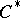-algebra). Such algebras are closely connected to important fields of geometric analysis, e.g., index theory, geometric quantization and several complex variables.

In the one-dimensional case one considers the Hardy spaceover the one-dimensional torus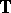(cf. also Hardy spaces), and defines the Toeplitz operator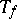with "symbol" functionby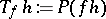for all, whereis the orthogonal projection given by the Cauchy integral theorem. The-algebragenerated by all operatorswith continuous symbolis not commutative, but defines a-algebra extensionof the-algebraof all compact operators; in fact, this "Toeplitz extension" is the generator of the Abelian group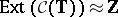.-algebra extensions are the building blocks of-theory and index theory; in our case a Toeplitz operatoris Fredholm (cf. also Fredholm operator) if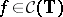has no zeros, and then the index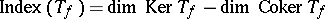is the (negative) winding number of.

In the multi-variable case, Toeplitz-algebras have been studied in several important cases, e.g. for strictly pseudo-convex domains[a1], including the unit ball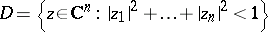[a2], [a10], for tube domains and Siegel domains over convex "symmetric" cones [a5], [a8], and for general bounded symmetric domains inhaving a transitive semi-simple Lie group of holomorphic automorphisms [a7]. Here, the principal new feature is the fact that Toeplitz operators(say, on the Hardy spaceover the Shilov boundaryof a pseudo-convex domain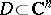) with continuous symbols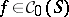are not essentially commuting, i.e.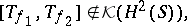in general. Thus, the corresponding Toeplitz-algebra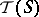is not a (one-step) extension of; instead one obtains a multi-step-filtration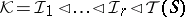of-ideals, with essentially commutative subquotients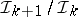, whose maximal ideal space (its spectrum) reflects the boundary strata of the underlying domain. The lengthof the composition series is an important geometric invariant, called the rank of. The index theory and-theory of these multi-variable Toeplitz-algebras is more difficult to study; on the other hand one obtains interesting classes of operators arising by geometric quantization of the underlying domain, regarded as a complex Kähler manifold.

A general method for studying the structure and representations of Toeplitz-algebras, at least for Shilov boundariesarising as a symmetric space (not necessarily Riemannian), is the so-called-duality [a11], [a9]. For example, ifis a Lie group with (reduced) group-algebra, then the so-called co-crossed product-algebra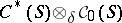induced by a natural co-actioncan be identified with. Now the Cauchy–Szegö orthogonal projection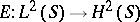(cf. also Cauchy operator) defines a certain-completion, and the corresponding Toeplitz-algebracan be realized as (a corner of)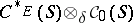. In this way the well-developed representation theory of (co-) crossed product-algebras [a4] can be applied to obtain Toeplitz-representations related to the boundary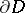. For example, the two-dimensional torus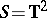gives rise to non-type--algebras (for cones with irrational slopes), and the underlying "Reinhardt" domains (cf. also Reinhardt domain) have interesting complex-analytic properties, such as a non-compact solution operator of the Neumann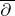-problem [a6].

How to Cite This Entry:
Toeplitz C*-algebra. Encyclopedia of Mathematics. URL: http://encyclopediaofmath.org/index.php?title=Toeplitz_C*-algebra&oldid=42916
This article was adapted from an original article by H. Upmeier (originator), which appeared in Encyclopedia of Mathematics - ISBN 1402006098. See original article